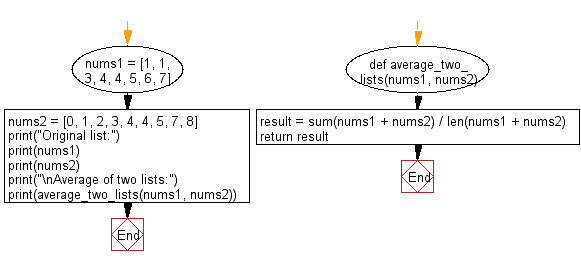﻿ Python: Compute average of two given lists - w3resource# Python: Compute average of two given lists

## Python List: Exercise - 105 with Solution

Write a Python program to compute average of two given lists.

Sample Solution:

Python Code:

``````def average_two_lists(nums1, nums2):
result = sum(nums1 + nums2) / len(nums1 + nums2)
return result

nums1 = [1, 1, 3, 4, 4, 5, 6, 7]
nums2 = [0, 1, 2, 3, 4, 4, 5, 7, 8]
print("Original list:")
print(nums1)
print(nums2)

print("\nAverage of two lists:")
print(average_two_lists(nums1, nums2))
```
```

Sample Output:

```Original list:
[1, 1, 3, 4, 4, 5, 6, 7]
[0, 1, 2, 3, 4, 4, 5, 7, 8]

Average of two lists:
3.823529411764706
```

Flowchart:## Visualize Python code execution:

The following tool visualize what the computer is doing step-by-step as it executes the said program:

Python Code Editor:

Have another way to solve this solution? Contribute your code (and comments) through Disqus.

What is the difficulty level of this exercise?

Test your Python skills with w3resource's quiz

﻿

## Python: Tips of the Day

Floor Division:

When we speak of division we normally mean (/) float division operator, this will give a precise result in float format with decimals.

For a rounded integer result there is (//) floor division operator in Python. Floor division will only give integer results that are round numbers.

```print(1000 // 300)
print(1000 / 300)```

Output:

```3
3.3333333333333335```